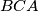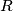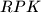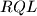### IMO Shortlist 2007 problem G1

Kvaliteta:
Avg: 3,0
Težina:
Avg: 6,0
In triangle$ABC$ the bisector of angle$BCA$ intersects the circumcircle again at$R$, the perpendicular bisector of$BC$ at$P$, and the perpendicular bisector of$AC$ at$Q$. The midpoint of$BC$ is$K$ and the midpoint of$AC$ is$L$. Prove that the triangles$RPK$ and$RQL$ have the same area.

Author: Marek Pechal, Czech Republic
Izvor: Međunarodna matematička olimpijada, shortlist 2007